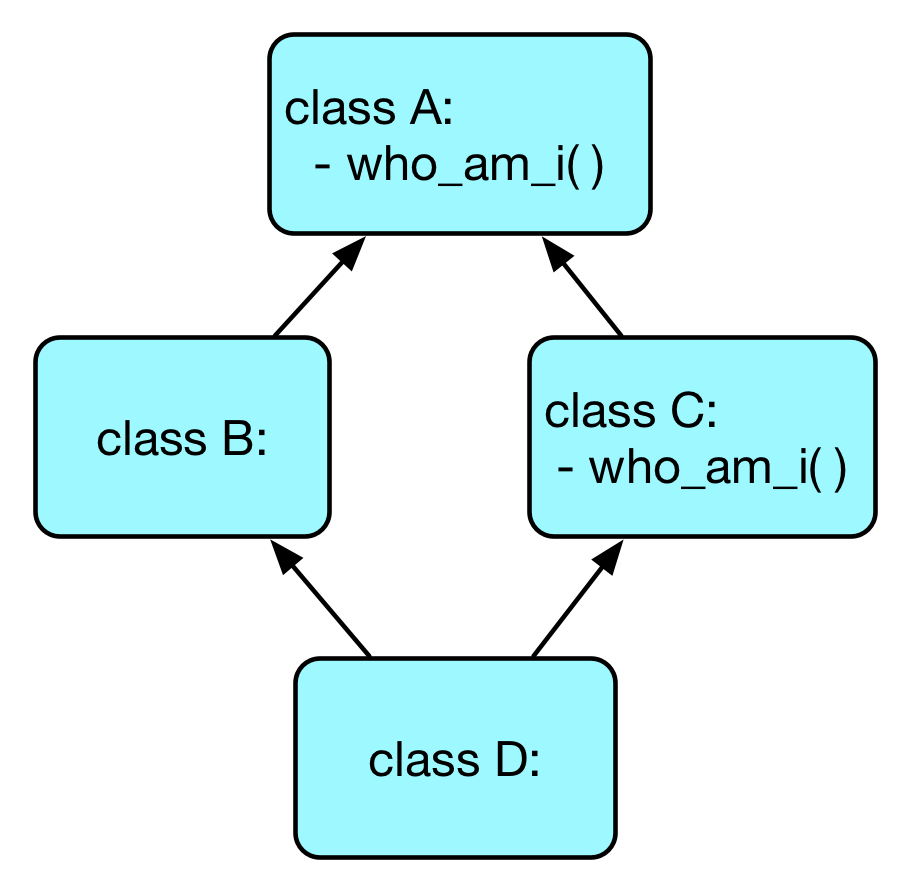# C3 线性化算法与 MRO

## 理解Python中的多继承

Posted by YuanBao on April 27, 2016

Python 中的方法解析顺序（Method Resolution Order, MRO）定义了多继承存在时 Python 解释器查找函数解析的正确方式。当 Python 版本从 2.2 发展到 2.3 再到现在的 Python 3，MRO算法也随之发生了相应的变化。这种变化在很多时候影响了我们使用不同版本 Python 编程的过程。

## 什么是 MRO

MRO 全称方法解析顺序（Method Resolution Order）。它定义了 Python 中多继承存在的情况下，解释器查找函数解析的具体顺序。什么是函数解析顺序？我们首先用一个简单的例子来说明。请仔细看下面代码：

class A():
def who_am_i(self):
print("I am A")

class B(A):
pass

class C(A):
def who_am_i(self):
print("I am C")

class D(B,C):
pass

d = D()


## New-style Class vs. Old-style Class

class A:
def __init__(self):
pass


Python 2.2 引入了新的模型对象（new-style class），其建议新的类型通过如下方式定义：

class A(object):
def __init__(self):
pass


## 理解 old-style class 的 MRO

1. 检查当前的类里面是否有该函数，如果有则直接调用。
2. 检查当前类的第一个父类里面是否有该函数，如果没有则检查父类的第一个父类是否有该函数，以此递归深度遍历。
3. 如果没有则回溯一层，检查下一个父类里面是否有该函数并按照 2 中的方式递归。>>> import inspect
>>> inspect.getmro(D)


## 理解 new-style class 的 MRO

>>> d.who_am_i()
I am C
>>> D.__mro__
(<class 'test.D'>, <class 'test.B'>, <class 'test.C'>, <class 'test.A'>, <class 'object'>)


## C3线性化算法

$head(C_{1}C_{2} \cdots C_{N})=C_{1}$ $tail(C_{1}C_{2} \cdots C_{N})=C_{2}C_{3} \cdots C_{N}$

$C_{1} + (C_{2}C_{3} \cdots C_{N}) = C_{1}C_{2} \cdots C_{N}$

$L[C(B_{1} \cdots B_{N})] = C + merge(L[B_{1}], \cdots, L[B_{N}], B_{1} \cdots B_{N})$

1. 选取 $merge$ 中的第一个列表记为当前列表 $K$。
2. 令 $h = head(K)$，如果 $h$ 没有出现在其他任何列表的 $tail$ 当中，那么将其加入到类 $C$ 的线性化列表中，并将其从 $merge$ 中所有列表中移除，之后重复步骤 2。
3. 否则，设置 $K$ 为 $merge$ 中的下一个列表，并重复 2 中的操作。
4. 如果 $merge$ 中所有的类都被移除，则输出类创建成功；如果不能找到下一个 $h$，则输出拒绝创建类 $C$ 并抛出异常。

class X():
def who_am_i(self):
print("I am a X")

class Y():
def who_am_i(self):
print("I am a Y")

class A(X, Y):
def who_am_i(self):
print("I am a A")

class B(Y, X):
def who_am_i(self):
print("I am a B")

class F(A, B):
def who_am_i(self):
print("I am a F")


$L[A] = A + merge[L[X], L[Y], X, Y] = [A, X, Y]$ $L[B] = B + merge[L[Y], L[X], Y, X] = [B, Y, X]$

$L[F] = F + merge[L[A], L[B], A, B] = F + merge[[A, X, Y], [B, Y, X], A, B]$

$L[F] = [F, A] + merge[[X, Y], [B, Y, X], B]$

$L[F] = [F, A, B] + merge[[X, Y], [Y, X]]$

Traceback (most recent call last):
File "test.py", line 17, in <module>
class F(A, B):
TypeError: Cannot create a consistent method resolution
order (MRO) for bases X, Y